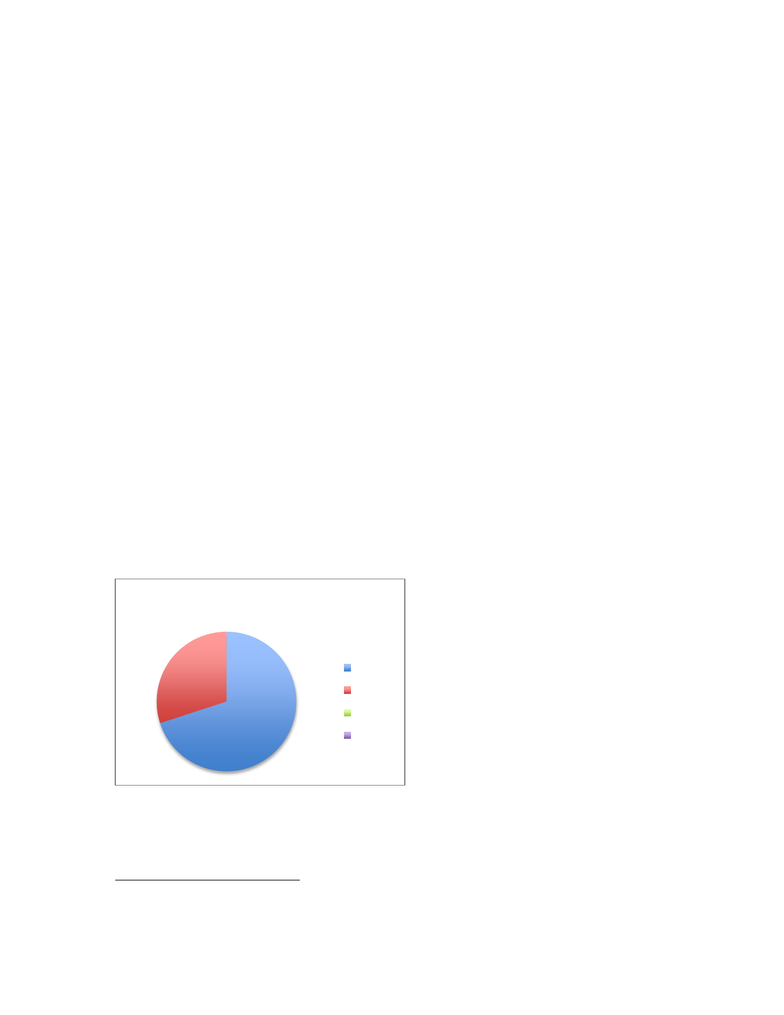Class Notes (1,100,000)
CA (650,000)
McGill (40,000)
ECON (100)
Lecture 4

# ECON 330D1 Lecture Notes - Lecture 4: Autarky, Production Function, Free Variables And Bound Variables

Department
Economics (Arts)
Course Code
ECON 330D1
Professor
Daniel Barczyk
Lecture
4

This preview shows half of the first page. to view the full 3 pages of the document.Econ 330 Lecture 4 September 18 2012
Y= F(K,L)
Production Function
1
+ Factors of Production
2
Economic Profit = Y MPL *L MPK * K
MPL *L= Total Wage MPK*K = Total Return
MPL = on graph equals w/p
CRS z*y
Because we are interested in the division of national income
Y= MPL *L +MPK *K + Economic profit
By Eulers theorem a CRS prod function can be written as Y= FL(K,L)=MPL*K+MPL*L
Economic profit are zero
In reality firms own capital, people own firms
Accounting profit = economic profit + mpk *k
The Cobb- Douglas production function
Observation: the division of national income between capital and labor had been
roughly constant over a long period of time
Constant factor shares capital income= y increases capital share of income
1
Output
2
K = Kbar, L= Lbar
Sales
Labor
Capital